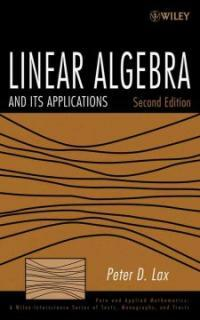> 상세정보

# 상세정보## Linear algebra and its applications 2nd ed (16회 대출)

자료유형
단행본
개인저자
Lax, Peter D. Lax, Peter D.
서명 / 저자사항
Linear algebra and its applications / Peter D. Lax.
판사항
2nd ed.
발행사항
Hoboken, N.J. :   John Wiley,   c2008.
형태사항
xv, 376 p. : ill. ; 25 cm.
총서사항
Pure and applied mathematics. A Wiley-Interscience of texts, monographs and tracts
ISBN
0471751561 9780471751564 (cloth)
일반주기
Previous ed.: Linear algebra. New York : Wiley, c1997.
내용주기
Fundamentals -- Duality -- Linear mappings -- Matrices -- Determinant and trace -- Spectral theory -- Euclidean structure -- Spectral theory of self-adjoint mappings -- Of a Euclidean space into itself -- Calculus of vector and matrix-valued functions -- Matrix inequalities -- Kinematics and dynamics -- Convexity -- The duality theorem -- Normed linear spaces -- Linear mappings between normed linear spaces -- Positive matrices -- How to solve systems of linear equations -- How to calculate the eigenvalues of self-adjoint matrices -- Solutions of selected exercises.
서지주기
Includes bibliographical references and index.
일반주제명
Algebras, Linear.
 000 01751camuu2200337 a 4500 001 000045419529 005 20110303185529 008 070601s2008 njua b 001 0 eng 010 ▼a 2007023226 020 ▼a 0471751561 020 ▼a 9780471751564 (cloth) 035 ▼a (KERIS)REF000013120430 040 ▼a DLC ▼c DLC ▼d DLC ▼d 211009 050 0 0 ▼a QA184.2 ▼b .L38 2008 082 0 0 ▼a 512/.5 ▼2 22 084 ▼a 512.5 ▼2 DDCK 090 ▼a 512.5 ▼b L4252L2 100 1 ▼a Lax, Peter D. 245 1 0 ▼a Linear algebra and its applications / ▼c Peter D. Lax. 250 ▼a 2nd ed. 260 ▼a Hoboken, N.J. : ▼b John Wiley, ▼c c2008. 300 ▼a xv, 376 p. : ▼b ill. ; ▼c 25 cm. 490 1 ▼a Pure and applied mathematics. A Wiley-Interscience of texts, monographs and tracts 500 ▼a Previous ed.: Linear algebra. New York : Wiley, c1997. 504 ▼a Includes bibliographical references and index. 505 0 ▼a Fundamentals -- Duality -- Linear mappings -- Matrices -- Determinant and trace -- Spectral theory -- Euclidean structure -- Spectral theory of self-adjoint mappings -- Of a Euclidean space into itself -- Calculus of vector and matrix-valued functions -- Matrix inequalities -- Kinematics and dynamics -- Convexity -- The duality theorem -- Normed linear spaces -- Linear mappings between normed linear spaces -- Positive matrices -- How to solve systems of linear equations -- How to calculate the eigenvalues of self-adjoint matrices -- Solutions of selected exercises. 650 0 ▼a Algebras, Linear. 700 1 ▼a Lax, Peter D. ▼t Linear algebra. 830 0 ▼a Pure and applied mathematics. ▼p A Wiley-Interscience of texts, monographs and tracts. 945 ▼a KINS

### 소장정보

No. 소장처 청구기호 등록번호 도서상태 반납예정일 예약 서비스
No. 1 소장처 청구기호 512.5 L4252L2 등록번호 121164303 도서상태 대출가능 반납예정일 예약 서비스
No. 2 소장처 청구기호 512.5 L4252L2 등록번호 121206509 도서상태 대출가능 반납예정일 예약 서비스

### 컨텐츠정보

#### 목차

`Preface.  Preface to the First Edition. 1. Fundamentals. 2. Duality. 3. Linear Mappings. 4. Matrices. 5. Determinant and Trace. 6. Spectral Theory. 7. Euclidean Structure. 8. Spectral Theory of Self-Adjoint Mappings. 9. Calculus of Vector- and Matrix-Valued Functions. 10. Matrix Inequalities. 11. Kinematics and Dynamics. 12. Convexity. 13. The Duality Theorem. 14. Normed Linear Spaces. 15. Linear Mappings Between Normed Linear Spaces. 16. Positive Matrices. 17. How to Solve Systems of Linear Equations. 18. How to Calculate the Eigenvalues of Self-Adjoint Matrices. 19. Solutions. Bibliography. Appendix 1. Special Determinants. Appendix 2. The Pfaffian. Appendix 3. Symplectic Matrices. Appendix 4. Tensor Product. Appendix 5. Lattices. Appendix 6. Fast Matrix Multiplication. Appendix 7. Gershgorin's Theorem. Appendix 8. The Multiplicity of Eigenvalues. Appendix 9. The Fast Fourier Transform. Appendix 10. The Spectral Radius. Appendix 11. The Lorentz Group. Appendix 12. Compactness of the Unit Ball. Appendix 13. A Characterization of Commutators. Appendix 14. Liapunov's Theorem. Appendix 15. The Jordan Canonical Form. Appendix 16. Numerical Range. Index.`

### 관련분야 신착자료

#### 정수론 첫걸음

Singh, Kuldeep (2022)

민만식 (2022)

엄정국 (2022)

#### 페르마의 마지막 정리 / 4판

Singh, Simon (2022)

강점란 (2022)

임근빈 (2022)

허걸 (2022)